# GAN生成对抗式神经网络实际操作

$V = E _ { x \sim P _ { \text {data} } } [ \log D ( x ) ] + E _ { x \sim P _ { G } } [ \log ( 1 - D ( x ) ) ]$

$\tilde { V } = \frac { 1 } { m } \sum _ { i = 1 } ^ { m } \log D \left( x ^ { i } \right) + \frac { 1 } { m } \sum _ { i = 1 } ^ { m } \log \left( 1 - D \left( \tilde { x } ^ { i } \right) \right)$

OK，分析完误差之后我们假定样本是十分给力的，那么我们就能根据面这道公式来做计算。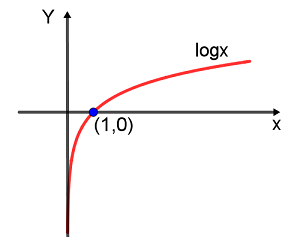$-\sum _ { i = 1 } ^ { m }p \left( x _ { i } \right)\log q \left( x _ { i } \right)-\sum _ { i = 1 } ^ { m }(1-p \left( x _ { i } \right))\log (1-q \left( \tilde { x } _ { i } \right))$

$-\sum _ { i = 1 } ^ { m }\log q \left( x _ { i } \right)-\sum _ { i = 1 } ^ { m }\log (1-q \left( \tilde { x } _ { i } \right))$

1.从$P _ { \text {data} }(x)$中抽出$m$个样本，写作$\left\{ x ^ { 1 } , x ^ { 2 } , \ldots , x ^ { m } \right\}$，再从$P _ { \text {G} }(x)$中抽出$m$个样本（也就是让$G$网络生成$m$个样本），写作$\left\{ \tilde { x } ^ { 1 } , \tilde { x } ^ { 2 } , \ldots , \tilde { x } ^ { m } \right\}$

2.用二分问题的交叉熵损失函数作为损失函数，然后用样本对网络进行训练，完事，就是这么简单。

$\tilde { V } = \frac { 1 } { m } \sum _ { i = 1 } ^ { m } \log D \left( x ^ { i } \right) + \frac { 1 } { m } \sum _ { i = 1 } ^ { m } \log \left( 1 - D \left( \tilde { x } ^ { i } \right) \right)$

$\tilde { V }_G= \frac { 1 } { m } \sum _ { i = 1 } ^ { m } \log \left( 1 - D \left( \tilde { x } ^ { i } \right) \right)$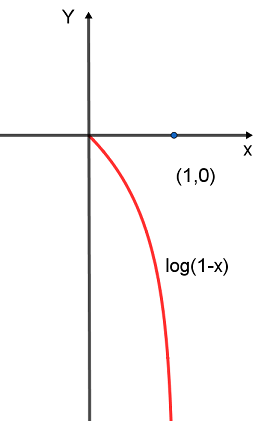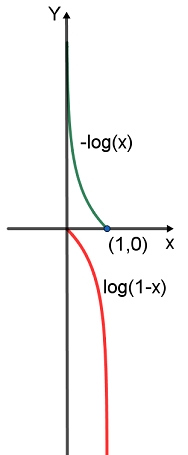$\tilde { V }_G= -\frac { 1 } { m } \sum _ { i = 1 } ^ { m } \log \left(D \left( \tilde { x } ^ { i } \right) \right)$

1.从$z$中抽出$m$个样本，写作$\left\{ \tilde { z } ^ { 1 } , \tilde { z } ^ { 2 } , \ldots , \tilde { z } ^ { m } \right\}$

2.用二分问题的交叉熵损失函数作为损失函数，然后用样本对网络进行训练，大功告成！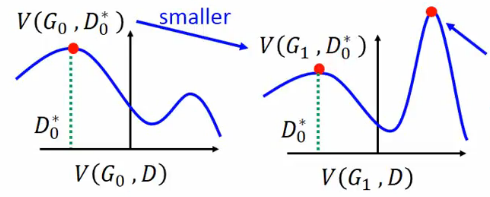model.py

# coding:utf-8
from torch import nn

class NetG(nn.Module):
"""
生成器定义
"""

def __init__(self, opt):
super(NetG, self).__init__()
ngf = opt.ngf  # 生成器feature map数

self.main = nn.Sequential(
# 输入是一个nz维度的噪声，我们可以认为它是一个1*1*nz的feature map
nn.ConvTranspose2d(opt.nz, ngf * 8, 4, 1, 0, bias=False),
nn.BatchNorm2d(ngf * 8),
nn.ReLU(True),
# 上一步的输出形状：(ngf*8) x 4 x 4

nn.ConvTranspose2d(ngf * 8, ngf * 4, 4, 2, 1, bias=False),
nn.BatchNorm2d(ngf * 4),
nn.ReLU(True),
# 上一步的输出形状： (ngf*4) x 8 x 8

nn.ConvTranspose2d(ngf * 4, ngf * 2, 4, 2, 1, bias=False),
nn.BatchNorm2d(ngf * 2),
nn.ReLU(True),
# 上一步的输出形状： (ngf*2) x 16 x 16

nn.ConvTranspose2d(ngf * 2, ngf, 4, 2, 1, bias=False),
nn.BatchNorm2d(ngf),
nn.ReLU(True),
# 上一步的输出形状：(ngf) x 32 x 32

nn.ConvTranspose2d(ngf, 3, 5, 3, 1, bias=False),
nn.Tanh()  # 输出范围 -1~1 故而采用Tanh
# 输出形状：3 x 96 x 96
)

def forward(self, input):
return self.main(input)

class NetD(nn.Module):
"""
判别器定义
"""

def __init__(self, opt):
super(NetD, self).__init__()
ndf = opt.ndf
self.main = nn.Sequential(
# 输入 3 x 96 x 96
nn.Conv2d(3, ndf, 5, 3, 1, bias=False),
nn.LeakyReLU(0.2, inplace=True),
# 输出 (ndf) x 32 x 32

nn.Conv2d(ndf, ndf * 2, 4, 2, 1, bias=False),
nn.BatchNorm2d(ndf * 2),
nn.LeakyReLU(0.2, inplace=True),
# 输出 (ndf*2) x 16 x 16

nn.Conv2d(ndf * 2, ndf * 4, 4, 2, 1, bias=False),
nn.BatchNorm2d(ndf * 4),
nn.LeakyReLU(0.2, inplace=True),
# 输出 (ndf*4) x 8 x 8

nn.Conv2d(ndf * 4, ndf * 8, 4, 2, 1, bias=False),
nn.BatchNorm2d(ndf * 8),
nn.LeakyReLU(0.2, inplace=True),
# 输出 (ndf*8) x 4 x 4

nn.Conv2d(ndf * 8, 1, 4, 1, 0, bias=False),
nn.Sigmoid()  # 输出一个数(概率)
)

def forward(self, input):
return self.main(input).view(-1)

main.py

# coding:utf-8
import os
import torch as t
import torchvision as tv
import tqdm
from model import NetG, NetD

class Config(object):
data_path = 'data/'  # 数据集存放路径
num_workers = 4  # 多进程加载数据所用的进程数
image_size = 96  # 图片尺寸
batch_size = 256  #一次训练样本数
max_epoch = 200  #最大训练次数
lr1 = 2e-4  # 生成器的学习率
lr2 = 2e-4  # 判别器的学习率
gpu = True  # 是否使用GPU
nz = 100  # 噪声维度
ngf = 64  # 生成器feature map数
ndf = 64  # 判别器feature map数

save_path = 'imgs/'  # 生成图片保存路径
d_every = 1  # 每1个batch训练一次判别器
g_every = 5  # 每5个batch训练一次生成器
save_every = 1  # 每1个epoch保存一次模型
#netd_path = 'checkpoints/netd.pth'
#netg_path = 'checkpoints/netg.pth'
netd_path = None
netg_path = None

opt = Config()

def train():
device=t.device('cuda') if opt.gpu else t.device('cpu')

# 读入数据格式转换
transforms = tv.transforms.Compose([
tv.transforms.Resize(opt.image_size),#图像尺寸缩放
tv.transforms.ToTensor(),
tv.transforms.Normalize((0.5, 0.5, 0.5), (0.5, 0.5, 0.5))#先将数据归一化到（0,1），再用公式(x-mean)/std将每个元素分布到（-1,1）
])

dataset = tv.datasets.ImageFolder(opt.data_path, transform=transforms)
batch_size=opt.batch_size,
shuffle=True,
num_workers=opt.num_workers,
drop_last=True
)

# 网络初始化，如有预训练模型则读入
netg, netd = NetG(opt), NetD(opt)
map_location = lambda storage, loc: storage
if opt.netd_path:
if opt.netg_path:
netd.to(device)
netg.to(device)

# 定义优化器和损失函数
optimizer_g = t.optim.Adam(netg.parameters(), opt.lr1, betas=(opt.beta1, 0.999))
optimizer_d = t.optim.Adam(netd.parameters(), opt.lr2, betas=(opt.beta1, 0.999))
criterion = t.nn.BCELoss().to(device)

# 真图片label为1，假图片label为0
# noises为生成网络的输入
true_labels = t.ones(opt.batch_size).to(device)
fake_labels = t.zeros(opt.batch_size).to(device)
fix_noises = t.randn(opt.batch_size, opt.nz, 1, 1).to(device)#产生正态分布的随机数，也就是G网络的z
noises = t.randn(opt.batch_size, opt.nz, 1, 1).to(device)

epochs = range(opt.max_epoch)
for epoch in iter(epochs):
for ii, (img, _) in tqdm.tqdm(enumerate(dataloader)):
real_img = img.to(device)

if ii % opt.d_every == 0:
# 训练判别器
## 尽可能的把真图片判别为正确
output = netd(real_img)
error_d_real = criterion(output, true_labels)
error_d_real.backward()

## 尽可能把假图片判别为错误
noises.data.copy_(t.randn(opt.batch_size, opt.nz, 1, 1))
fake_img = netg(noises).detach()  # 根据噪声生成假图
output = netd(fake_img)
error_d_fake = criterion(output, fake_labels)
error_d_fake.backward()
optimizer_d.step()

if ii % opt.g_every == 0:
# 训练生成器
noises.data.copy_(t.randn(opt.batch_size, opt.nz, 1, 1))
fake_img = netg(noises)
output = netd(fake_img)
error_g = criterion(output, true_labels)
error_g.backward()
optimizer_g.step()

if (epoch+1) % opt.save_every == 0:
# 保存模型、图片
fix_fake_imgs = netg(fix_noises)
tv.utils.save_image(fix_fake_imgs.data[:64], '%s/%s.png' % (opt.save_path, epoch), normalize=True,range=(-1, 1))
t.save(netd.state_dict(), 'checkpoints/netd.pth')
t.save(netg.state_dict(), 'checkpoints/netg.pth')
t.save(netd.state_dict(), 'checkpoints/netd_%s.pth' % epoch)
t.save(netg.state_dict(), 'checkpoints/netg_%s.pth' % epoch)

if __name__ == '__main__':
train()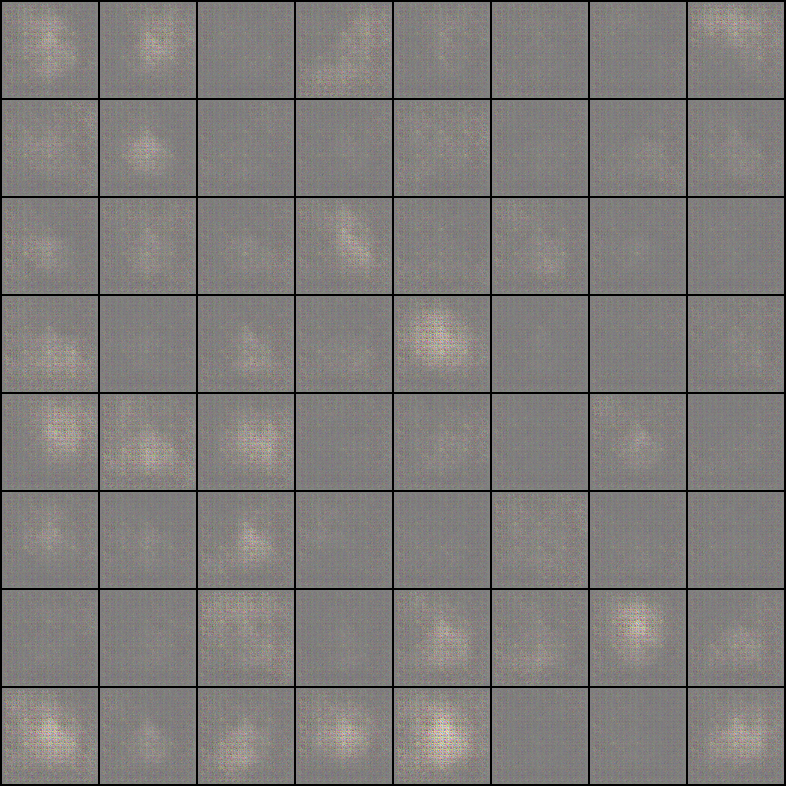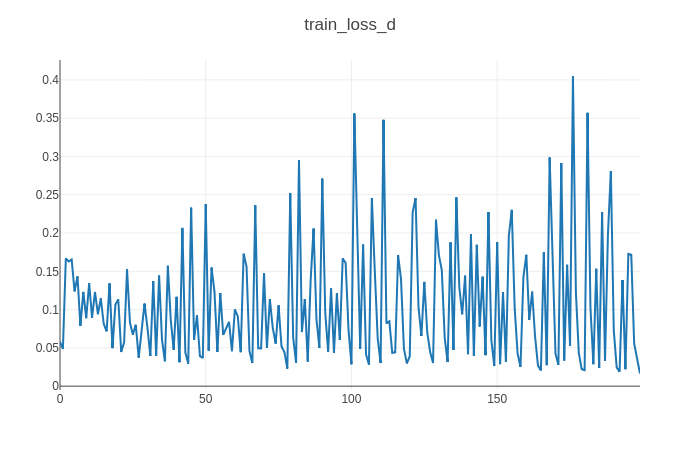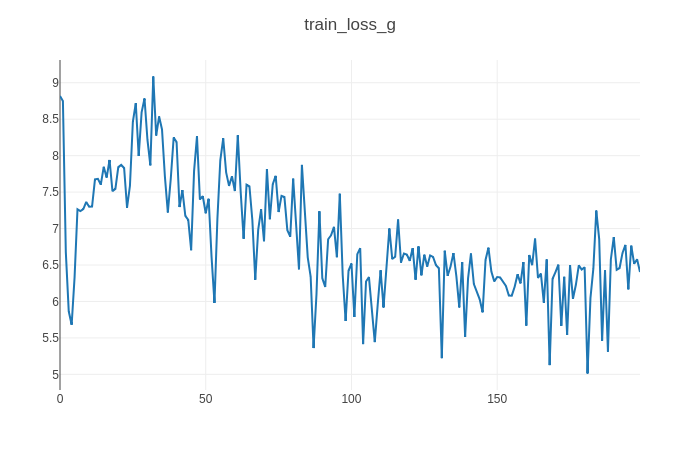# coding:utf-8
import os
import torch as t
import torchvision as tv
import tqdm
from model import NetG, NetD
import visdom

class Config(object):
data_path = 'data/'  # 数据集存放路径
num_workers = 4  # 多进程加载数据所用的进程数
image_size = 96  # 图片尺寸
batch_size = 256  #一次训练样本数
max_epoch = 200  #最大训练次数
lr1 = 2e-4  # 生成器的学习率
lr2 = 2e-4  # 判别器的学习率
gpu = True  # 是否使用GPU
nz = 100  # 噪声维度
ngf = 64  # 生成器feature map数
ndf = 64  # 判别器feature map数

save_path = 'imgs/'  # 生成图片保存路径
d_every = 1  # 每1个batch训练一次判别器
g_every = 5  # 每5个batch训练一次生成器
save_every = 1  # 每1个epoch保存一次模型
#netd_path = 'checkpoints/netd.pth'
#netg_path = 'checkpoints/netg.pth'
netd_path = None
netg_path = None

opt = Config()

def train():
device=t.device('cuda') if opt.gpu else t.device('cpu')

# 读入数据格式转换
transforms = tv.transforms.Compose([
tv.transforms.Resize(opt.image_size),#图像尺寸缩放
tv.transforms.ToTensor(),
tv.transforms.Normalize((0.5, 0.5, 0.5), (0.5, 0.5, 0.5))#先将数据归一化到（0,1），再用公式(x-mean)/std将每个元素分布到（-1,1）
])

dataset = tv.datasets.ImageFolder(opt.data_path, transform=transforms)
batch_size=opt.batch_size,
shuffle=True,
num_workers=opt.num_workers,
drop_last=True
)

# 网络初始化，如有预训练模型则读入
netg, netd = NetG(opt), NetD(opt)
map_location = lambda storage, loc: storage
if opt.netd_path:
if opt.netg_path:
netd.to(device)
netg.to(device)

# 定义优化器和损失函数
optimizer_g = t.optim.Adam(netg.parameters(), opt.lr1, betas=(opt.beta1, 0.999))
optimizer_d = t.optim.Adam(netd.parameters(), opt.lr2, betas=(opt.beta1, 0.999))
criterion = t.nn.BCELoss().to(device)

# 真图片label为1，假图片label为0
# noises为生成网络的输入
true_labels = t.ones(opt.batch_size).to(device)
fake_labels = t.zeros(opt.batch_size).to(device)
fix_noises = t.randn(opt.batch_size, opt.nz, 1, 1).to(device)#产生正态分布的随机数，也就是G网络的z
noises = t.randn(opt.batch_size, opt.nz, 1, 1).to(device)

#可视化
vis = visdom.Visdom()

epochs = range(opt.max_epoch)
for epoch in iter(epochs):
for ii, (img, _) in tqdm.tqdm(enumerate(dataloader)):
real_img = img.to(device)

if ii % opt.d_every == 0:
# 训练判别器
## 尽可能的把真图片判别为正确
output = netd(real_img)
error_d_real = criterion(output, true_labels)
error_d_real.backward()

## 尽可能把假图片判别为错误
noises.data.copy_(t.randn(opt.batch_size, opt.nz, 1, 1))
fake_img = netg(noises).detach()  # 根据噪声生成假图
output = netd(fake_img)
error_d_fake = criterion(output, fake_labels)
error_d_fake.backward()
optimizer_d.step()

if ii % opt.g_every == 0:
# 训练生成器
noises.data.copy_(t.randn(opt.batch_size, opt.nz, 1, 1))
fake_img = netg(noises)
output = netd(fake_img)
error_g = criterion(output, true_labels)
error_g.backward()
optimizer_g.step()

if (epoch+1) % opt.save_every == 0:
# 保存模型、图片
fix_fake_imgs = netg(fix_noises)
tv.utils.save_image(fix_fake_imgs.data[:64], '%s/%s.png' % (opt.save_path, epoch), normalize=True,range=(-1, 1))
vis.images(fix_fake_imgs.detach().cpu().numpy()[:64] * 0.5 + 0.5, win='fixfake')
t.save(netd.state_dict(), 'checkpoints/netd.pth')
t.save(netg.state_dict(), 'checkpoints/netg.pth')
t.save(netd.state_dict(), 'checkpoints/netd_%s.pth' % epoch)
t.save(netg.state_dict(), 'checkpoints/netg_%s.pth' % epoch)

if __name__ == '__main__':
train()

GAN生成对抗式神经网络实际操作

### 手机扫码访问

•